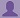SHARE
HELP

To start with, Sr2Jr’s first step is to reduce the expenses related to education. To achieve this goal Sr2Jr organized the textbook’s question and answers. Sr2Jr is community based and need your support to fill the question and answers. The question and answers posted will be available free of cost to all.# Computer Networking : A Top-down Approach

Authors:
James F. Kurose, Keith W. Ross
Exercise:
Problems
Chapter:
Transport Layer
Edition:
6
ISBN:
9780132856201
Question:
31
 Previous Next

###Question

Suppose that the five measured SampleRTT values (see Section 3.5.3) are 106 ms, 120 ms, 140 ms, 90 ms, and 115 ms. Compute the EstimatedRTT after each of these SampleRTT values is obtained, using a value of α = 0.125 and assuming that the value of EstimatedRTT was 100 ms just before the first of these five samples were obtained. Compute also the DevRTT after each sample is obtained, assuming a value of β = 0.25 and assuming the value of DevRTT was 5 ms just before the first of these five samples was obtained. Last, compute the TCP TimeoutInterval after each of these samples is obtained.

###Answer

Calculate the EstimatedRTT after obtaining the first sample RTT=106ms,

EstimatedRTT = α * SampleRTT+(1- α) * EstimatedRTT

EstimatedRTT =0.125 * 106 + (1-0.125) * 100

=0.125* 106 + 0.875 * 100

=13.25 + 87.5

=100.75ms

Calculate the DevRTT after obtaining the first sample RTT:

DevRTT = β * | SampleRTT- EstimatedRTT|+(1- β)* DevRTT

=0.25 * |106-100.75| + (1-0.25) *5

=0.25 *5.25 + 0.75 * 5

=1.3125 + 3.75

=5.0625ms

Calculate the Timeout Interval after obtaining the first sample RTT:

TimeoutInterval = EstimatedRTT +4* DevRTT

= 100.75 + 4 *5.0625

=121ms

Calculate the EstimatedRTT after obtaining the second sample RTT=120ms,

EstimatedRTT = α * SampleRTT+(1- α) * EstimatedRTT

EstimatedRTT =0.125 * 120 + (1-0.125) * 100.75

=0.125* 120 + 0.875 * 100.75

=15 + 88.15625

=103.15625ms

Calculate the DevRTT after obtaining the second sample RTT:

DevRTT = β * | SampleRTT- EstimatedRTT|+(1- β)* DevRTT

=0.25 * |120-103.15625| + (1-0.25) *5.0625

=0.25 *16.84 + 0.75 * 5.0625

=4.21 + 3.79

=8ms

Calculate the Timeout Interval after obtaining the second sample RTT:

TimeoutInterval = EstimatedRTT +4* DevRTT

= 103.15 + 4 *8

=135.15ms

Calculate the EstimatedRTT after obtaining the third sample RTT=140ms:

EstimatedRTT = α * SampleRTT+(1- α) * EstimatedRTT

EstimatedRTT =0.125 * 140 + (1-0.125) * 103.15

=0.125* 140 + 0.875 * 103.15

=17.5 +90.26

=107.75ms

Calculate the DevRTT after obtaining the third sample RTT:

DevRTT = β * | SampleRTT- EstimatedRTT|+(1- β)* DevRTT

=0.25 * |140-107.75| + (1-0.25) *8

=0.25 *32.25 + 0.75 * 8

=8.06 + 6

=14.06ms

Calculate the Timeout Interval after obtaining the third sample RTT:

TimeoutInterval = EstimatedRTT +4* DevRTT

= 107.75 + 4 *14.06

=164ms

Calculate the EstimatedRTT after obtaining the fourth sample RTT=90ms:

EstimatedRTT = α * SampleRTT+(1- α) * EstimatedRTT

EstimatedRTT =0.125 * 90 + (1-0.125) * 107.75

=0.125* 90 + 0.875 * 107.75

=11.25 +94.28

=105.53ms

Calculate the DevRTT after obtaining the fourth sample RTT:

DevRTT = β * | SampleRTT- EstimatedRTT|+(1- β)* DevRTT

=0.25 * |90-105.53| + (1-0.25) *14.06

=0.25 *15.53 + 0.75 * 14.06

=3.88 + 10.545

=14.42ms

Calculate the Timeout Interval after obtaining the fourth sample RTT:

TimeoutInterval = EstimatedRTT +4* DevRTT

= 105.53 + 4 *14.42

=163.21ms

Calculate the EstimatedRTT after obtaining the fifth sample RTT=115ms:

EstimatedRTT = α * SampleRTT+(1- α) * EstimatedRTT

EstimatedRTT =0.125 * 115 + (1-0.125) * 105.53

=0.125* 115 + 0.875 * 105.53

=14.375 +92.34

=106.715ms

Calculate the DevRTT after obtaining the fifth sample RTT:

DevRTT = β * | SampleRTT- EstimatedRTT|+(1- β)* DevRTT

=0.25 * |115-106.715| + (1-0.25) *14.42

=0.25 *8.285 + 0.75 * 14.42

=2.07 + 10.815

=12.885ms

Calculate the Timeout Interval after obtaining the fifth sample RTT:

TimeoutInterval = EstimatedRTT +4* DevRTT

= 106.715 + 4 *12.885

=158.255ms

### Discussions

Post the discussion to improve the above solution.